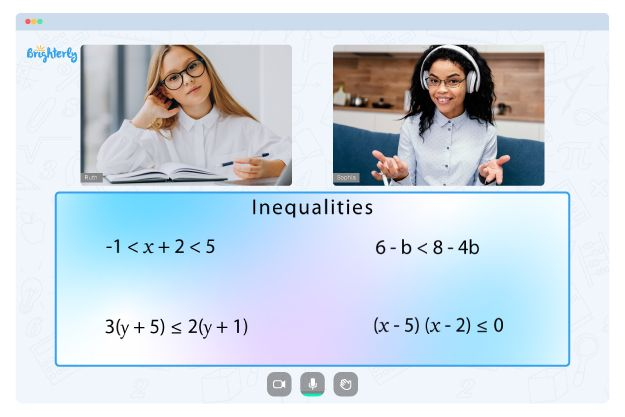# Greater Than or Equal to

Welcome to another captivating post on Brighterly, the go-to destination for children who are eager to learn, discover, and excel in the captivating realm of mathematics! Our topic today is Greater Than or Equal to – a fundamental concept that young learners need to comprehend to unlock their true mathematical potential. So, come along, and let’s embark on this exciting learning adventure filled with creativity, fun, and interaction.

## What Does Greater Than or Equal to Mean?

The term “Greater Than or Equal to” refers to a comparison between two numbers, values, or quantities. When we say that one number is greater than or equal to another, it implies that the first number is either larger than the second number or equal to it. We use the symbol “≥” to represent this relationship. There are three basic symbols used for comparison:

### Less than (<):

The less than symbol (<) is used when one number is smaller than another. For example, 3 < 5, meaning that 3 is less than 5.

### Greater than (>):

The greater than symbol (>) is used when one number is larger than another. For example, 7 > 2, meaning that 7 is greater than 2.

### Greater than or Equal to (≥):

The greater than or equal to symbol (≥) is used when one number is either larger than or equal to another. For example, 5 ≥ 3, meaning that 5 is greater than or equal to 3.

## Examples of Greater than or Equal to

Here are a few examples of greater than or equal to:

1. 8 ≥ 5: In this example, the number 8 is greater than the number 5.
2. 3 ≥ 3: In this example, the number 3 is equal to the number 3.

## Non Examples of Greater than or Equal to

Here are a few non-examples of greater than or equal to:

1. 4 ≥ 9: In this example, the number 4 is not greater than or equal to the number 9.
2. 2 ≥ 6: In this example, the number 2 is not greater than or equal to the number 6.

## Greater than or Equal to on a Number line:

A number line is a helpful tool for visualizing the concept of greater than or equal to. If two points on the number line are equal or the first point is to the right of the second point, the first number is greater than or equal to the second number.

## Difference between Comparison Symbols:

It is crucial to understand the difference between the three comparison symbols, as they convey different relationships between numbers. The less than symbol (<) shows a smaller value, the greater than symbol (>) shows a larger value, and the greater than or equal to symbol (≥) shows either a larger or equal value.

## Solved Examples

1. Is 7 ≥ 4? Yes, because 7 is greater than 4.
2. Is 5 ≥ 5? Yes, because 5 is equal to 5.
3. Is 3 ≥ 8? No, because 3 is not greater than or equal to 8.

1. Is 6 ≥ 3?
2. Is 9 ≥ 11?
3. Is 4 ≥ 4?

## Conclusion

By thoroughly grasping the concept of greater than or equal to and acquiring proficiency in utilizing comparison symbols, children can truly flourish in the world of mathematics and elevate their problem-solving abilities. Remember, practice makes perfect! With consistent practice and the right guidance, mathematical concepts will become second nature. And as always, Brighterly is your steadfast partner in transforming math learning into an enthralling and enjoyable experience for young minds. We’re here to support and inspire you every step of the way in your journey to become a math whiz!

## Frequently Asked Questions on Greater Than or Equal to

### How can I help my child understand greater than or equal to?

1. Use real-life examples, such as comparing the number of toys or items of clothing they have.
2. Use visual aids, like a number line, to show the position of numbers and their relationship.
3. Practice and reinforce the concept with worksheets and interactive activities.

### What are the other comparison symbols in math?

In addition to greater than or equal to (≥), other comparison symbols in math include:

1. Less than (<)
2. Greater than (>)
3. Less than or equal to (≤)
4. Equal to (=)

### Can greater than or equal to be used with fractions and decimals?

Yes, greater than or equal to can be used with fractions and decimals as well. For example:

1. 1/2 ≥ 1/4: The fraction 1/2 is greater than or equal to 1/4.
2. 0.5 ≥ 0.4: The decimal 0.5 is greater than or equal to 0.4.
Information Sources

For more information on the topic of Greater Than or Equal to, you can refer to the following sources:

Struggling with Inequalities?• Is your child having difficulties with understanding the fundamentals of inequalities?
• An online tutor could provide the necessary guidance.

Does your child need additional help with mastering inequalities lessons? Start lessons with an online tutor.